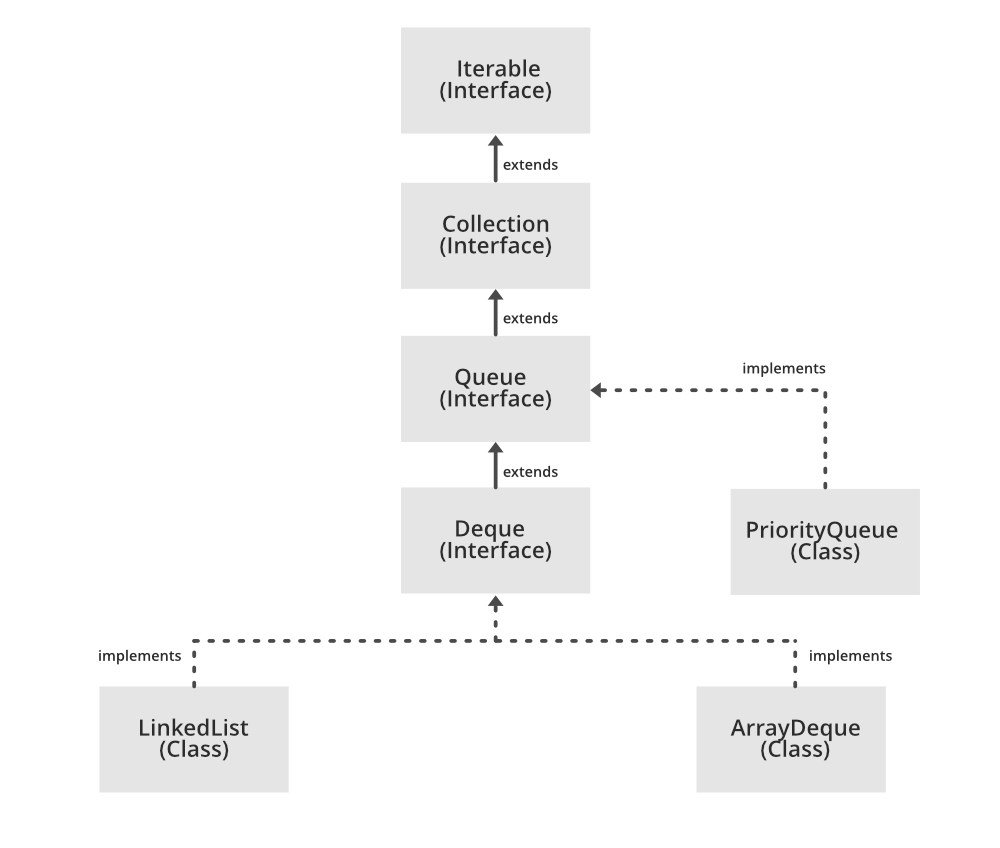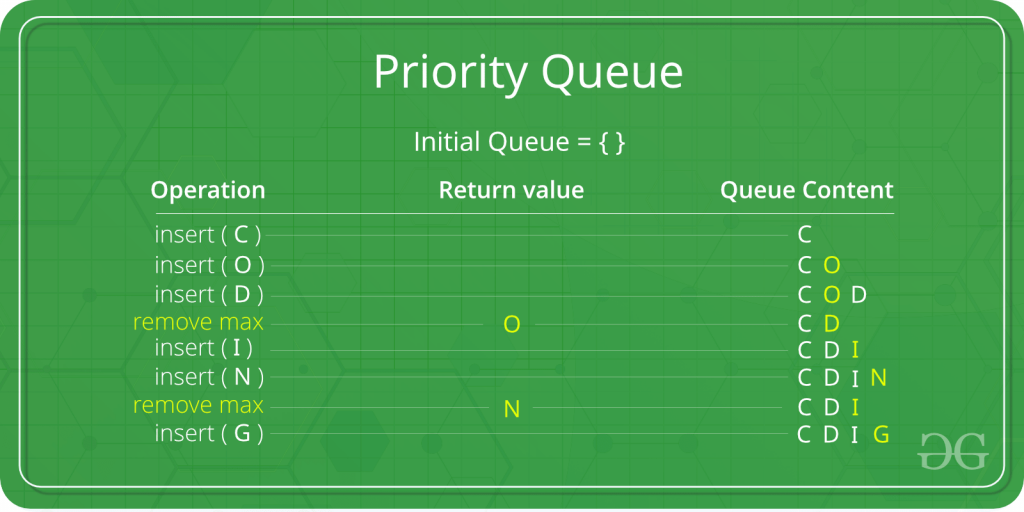# PriorityQueue in Java

• Difficulty Level : Medium
• Last Updated : 18 May, 2022

A PriorityQueue is used when the objects are supposed to be processed based on the priority. It is known that a Queue follows the First-In-First-Out algorithm, but sometimes the elements of the queue are needed to be processed according to the priority, that’s when the PriorityQueue comes into play.The PriorityQueue is based on the priority heap. The elements of the priority queue are ordered according to the natural ordering, or by a Comparator provided at queue construction time, depending on which constructor is used.In the below priority queue, an element with a maximum ASCII value will have the highest priority.Declaration:

```public class PriorityQueue<E> extends AbstractQueue<E> implements Serializable

where E is the type of elements held in this queue```

The class implements Serializable, Iterable<E>, Collection<E>, Queue<E> interfaces.

A few important points on Priority Queue are as follows:

• PriorityQueue doesn’t permit null.
• We can’t create a PriorityQueue of Objects that are non-comparable
• PriorityQueue are unbound queues.
• The head of this queue is the least element with respect to the specified ordering. If multiple elements are tied for the least value, the head is one of those elements — ties are broken arbitrarily.
• Since PriorityQueue is not thread-safe, java provides PriorityBlockingQueue class that implements the BlockingQueue interface to use in a java multithreading environment.
• The queue retrieval operations poll,  remove,  peek, and element access the element at the head of the queue.
• It provides O(log(n)) time for add and poll methods.
• It inherits methods from AbstractQueue, AbstractCollection, Collection, and Object class.

Constructors:

1. PriorityQueue(): Creates a PriorityQueue with the default initial capacity (11) that orders its elements according to their natural ordering.

PriorityQueue<E> pq = new PriorityQueue<E>();

2. PriorityQueue(Collection<E> c): Creates a PriorityQueue containing the elements in the specified collection.

PriorityQueue<E> pq = new PriorityQueue<E>(Collection<E> c);

3. PriorityQueue(int initialCapacity): Creates a PriorityQueue with the specified initial capacity that orders its elements according to their natural ordering.

PriorityQueue<E> pq = new PriorityQueue<E>(int initialCapacity);

4. PriorityQueue(int initialCapacity, Comparator<E> comparator): Creates a PriorityQueue with the specified initial capacity that orders its elements according to the specified comparator.

PriorityQueue<E> pq = new PriorityQueue(int initialCapacity, Comparator<E> comparator);

5. PriorityQueue(PriorityQueue<E> c): Creates a PriorityQueue containing the elements in the specified priority queue.

PriorityQueue<E> pq = new PriorityQueue(PriorityQueue<E> c);

6. PriorityQueue(SortedSet<E> c): Creates a PriorityQueue containing the elements in the specified sorted set.

PriorityQueue<E> pq = new PriorityQueue<E>(SortedSet<E> c);

Example:

The example below explains the following basic operations of the priority queue.

• boolean add(E element): This method inserts the specified element into this priority queue.
• public peek(): This method retrieves, but does not remove, the head of this queue, or returns null if this queue is empty.
• public poll(): This method retrieves and removes the head of this queue, or returns null if this queue is empty.

## Java

 `// Java program to demonstrate the ` `// working of PriorityQueue ` `import` `java.util.*; ` ` `  `class` `PriorityQueueDemo { ` `   `  `      ``// Main Method ` `    ``public` `static` `void` `main(String args[]) ` `    ``{ ` `        ``// Creating empty priority queue ` `        ``PriorityQueue pQueue = ``new` `PriorityQueue(); ` ` `  `        ``// Adding items to the pQueue using add() ` `        ``pQueue.add(``10``); ` `        ``pQueue.add(``20``); ` `        ``pQueue.add(``15``); ` ` `  `        ``// Printing the top element of PriorityQueue ` `        ``System.out.println(pQueue.peek()); ` ` `  `        ``// Printing the top element and removing it ` `        ``// from the PriorityQueue container ` `        ``System.out.println(pQueue.poll()); ` ` `  `        ``// Printing the top element again ` `        ``System.out.println(pQueue.peek()); ` `    ``} ` `} `

Output

```10
10
15```

### Operations on PriorityQueue

Let’s see how to perform a few frequently used operations on the Priority Queue class.

1. Adding Elements: In order to add an element in a priority queue, we can use the add() method. The insertion order is not retained in the PriorityQueue. The elements are stored based on the priority order which is ascending by default.

## Java

 `/*package whatever //do not write package name here */` ` `  `import` `java.util.*; ` `import` `java.io.*; ` `   `  `public` `class` `PriorityQueueDemo { ` `   `  `    ``public` `static` `void` `main(String args[]) ` `    ``{ ` `        ``PriorityQueue pq = ``new` `PriorityQueue<>(); ` `        ``for``(``int` `i=``0``;i<``3``;i++){ ` `            ``pq.add(i); ` `            ``pq.add(``1``); ` `        ``} ` `        ``System.out.println(pq); ` `    ``} ` `} `

Output

`[0, 1, 1, 1, 2, 1]`

We will not get sorted elements by printing PriorityQueue.

## Java

 `/*package whatever //do not write package name here */` ` `  `import` `java.util.*; ` `import` `java.io.*; ` `   `  `public` `class` `PriorityQueueDemo { ` `   `  `    ``public` `static` `void` `main(String args[]) ` `    ``{ ` `        ``PriorityQueue pq = ``new` `PriorityQueue<>(); ` `   `  `        ``pq.add(``"Geeks"``); ` `        ``pq.add(``"For"``); ` `        ``pq.add(``"Geeks"``); ` `   `  `        ``System.out.println(pq); ` `    ``} ` `}`

Output

`[For, Geeks, Geeks]`

2. Removing Elements: In order to remove an element from a priority queue, we can use the remove() method. If there are multiple such objects, then the first occurrence of the object is removed. Apart from that, the poll() method is also used to remove the head and return it.

## Java

 `// Java program to remove elements ` `// from a PriorityQueue ` ` `  `import` `java.util.*; ` `import` `java.io.*; ` ` `  `public` `class` `PriorityQueueDemo { ` ` `  `    ``public` `static` `void` `main(String args[]) ` `    ``{ ` `        ``PriorityQueue pq = ``new` `PriorityQueue<>(); ` ` `  `        ``pq.add(``"Geeks"``); ` `        ``pq.add(``"For"``); ` `        ``pq.add(``"Geeks"``); ` ` `  `        ``System.out.println(``"Initial PriorityQueue "` `+ pq); ` ` `  `          ``// using the method ` `        ``pq.remove(``"Geeks"``); ` ` `  `        ``System.out.println(``"After Remove - "` `+ pq); ` ` `  `        ``System.out.println(``"Poll Method - "` `+ pq.poll()); ` ` `  `        ``System.out.println(``"Final PriorityQueue - "` `+ pq); ` `    ``} ` `}`

Output

```Initial PriorityQueue [For, Geeks, Geeks]
After Remove - [For, Geeks]
Poll Method - For
Final PriorityQueue - [Geeks]```

3. Accessing the elements: Since Queue follows the First In First Out principle, we can access only the head of the queue. To access elements from a priority queue, we can use the peek() method.

## Java

 `// Java program to access elements ` `// from a PriorityQueue ` `import` `java.util.*; ` ` `  `class` `PriorityQueueDemo { ` `   `  `      ``// Main Method ` `    ``public` `static` `void` `main(String[] args) ` `    ``{ ` ` `  `        ``// Creating a priority queue ` `        ``PriorityQueue pq = ``new` `PriorityQueue<>(); ` `        ``pq.add(``"Geeks"``); ` `        ``pq.add(``"For"``); ` `        ``pq.add(``"Geeks"``); ` `        ``System.out.println(``"PriorityQueue: "` `+ pq); ` ` `  `        ``// Using the peek() method ` `        ``String element = pq.peek(); ` `        ``System.out.println(``"Accessed Element: "` `+ element); ` `    ``} ` `}`

Output

```PriorityQueue: [For, Geeks, Geeks]
Accessed Element: For```

4. Iterating the PriorityQueue: There are multiple ways to iterate through the PriorityQueue. The most famous way is converting the queue to the array and traversing using the for loop. However, the queue also has an inbuilt iterator which can be used to iterate through the queue.

## Java

 `// Java program to iterate elements ` `// to a PriorityQueue ` ` `  `import` `java.util.*; ` ` `  `public` `class` `PriorityQueueDemo { ` ` `  `      ``// Main Method ` `    ``public` `static` `void` `main(String args[]) ` `    ``{ ` `        ``PriorityQueue pq = ``new` `PriorityQueue<>(); ` ` `  `        ``pq.add(``"Geeks"``); ` `        ``pq.add(``"For"``); ` `        ``pq.add(``"Geeks"``); ` ` `  `        ``Iterator iterator = pq.iterator(); ` ` `  `        ``while` `(iterator.hasNext()) { ` `            ``System.out.print(iterator.next() + ``" "``); ` `        ``} ` `    ``} ` `}`

Output

`For Geeks Geeks `

Applications

Related Articles